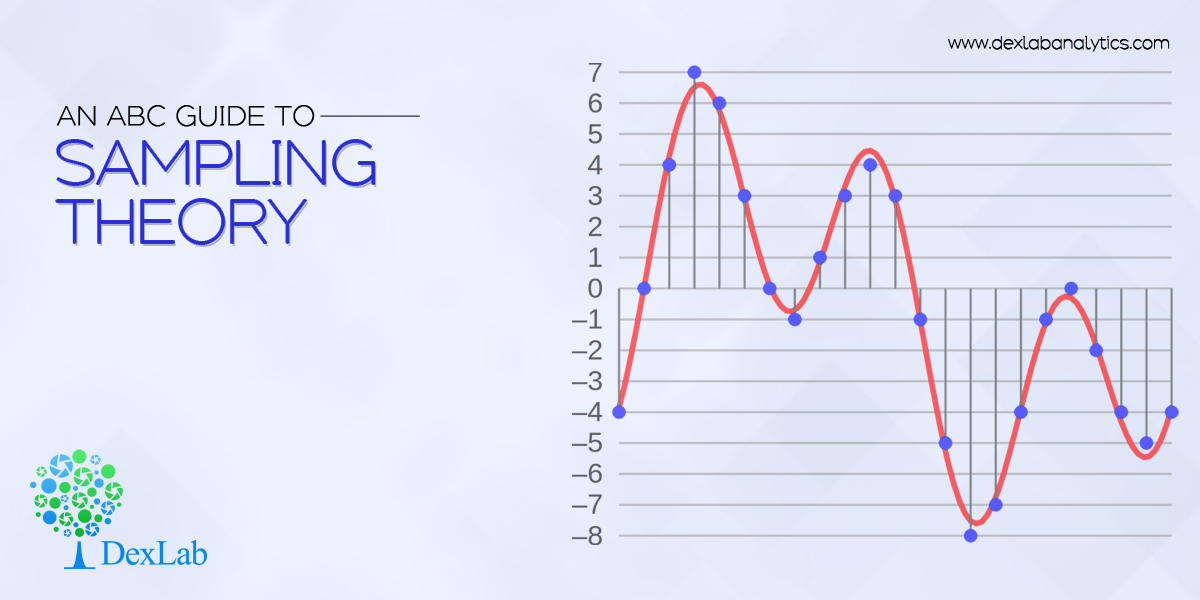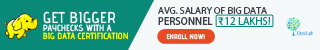An ABC Guide to Sampling Theory

# An ABC Guide to Sampling TheorySampling theory is a study involving collection, analysis and interpretation of data accumulated from random samples of a population. It’s a separate branch of statistics that observes the relationship existing between a population and samples drawn from the population.

In simple terms, sampling means the procedure of drawing a sample out of a population. It aids us to draw a conclusion about the characteristics of the population after carefully studying only the objects present in the sample.

Here we’ve whisked out a few sampling-related terms and their definitions that would help you understand the nuanced notion of sampling better. Let’s have a look:

Sample – It’s the finite representative subset of a population. It’s chosen from a population with an aim to scrutiny its properties and principles.

Population – When a statistical investigation focuses on the study of numerous characteristics involving items on individuals associated with a particular group, this group under study is known as the population or the universe. A group containing a finite number of objects is known as finite population, while a group with infinite or large number of objects is called infinite population.

Population parameter – It’s an obscure numerical factor of the population. It’s no brainer that the primary objective of a survey is to find the values of different measures of population distribution; and the parameters are nothing but a functional variant inclusive of all population units.Estimator – Calculated based on sample values, an estimator is a functional measure.

Sampling fluctuation of an estimator – When you draw a particular sample from a given population, it contains different set of population members. As a result, the value of the estimator varies from one sample to another. This difference in values of the estimator is known as the sampling fluctuations of an estimator.

Next, we would like to discuss about the types of sampling:

There are mainly two types of random sampling, and they are as follows:

#### Simple Random Sampling with Replacement

In the first case, the ‘n’ units of the sample are drawn from the population in such a way that at each drawing, each of the ‘n’ numbers of the population gets the same probability 1⁄N of being selected. Hence, this methods is called the simple random sampling with replacement, clearly, the same unit of population may occur more than once inj a simple. Hence, there are N^n samples, regard being to the orders in which ‘n’ sample unit occur and each such sample has the probability 1/N^n .

#### Simple Random Sampling Without Replacement

In the second case each of the ‘n’ members of the sample are drawn one by one but the members once drawn are not returned back to the population and at each stage remaining amount of the population is given the same probability of being includes in the sample. This method of drawing the sample is called SRSWOR therefore under SRSWOR at any r^th number of draw there remains (N-r+1) units. And each unit has the probability of 1/((N-r+1) ) of being drawn.

Remember, if we take ‘n’ individuals at once from a given population giving equal probability to each of the observations, then the total number of possible example in (_n^N)C i.e.., combination of ‘n’ members out of ‘N’ numbers of the population will from the total no. of possible sample in SRSWOR.

The world of statistics is huge and intensively challenging. And so is sampling theory.

But, fret now. Our data science courses in Noida will help you understand the nuances of this branch of statistics. For more, visit our official site.

P.S: This is our first blog of the series ‘sampling theory’. The rest will follow soon. Stay tuned.